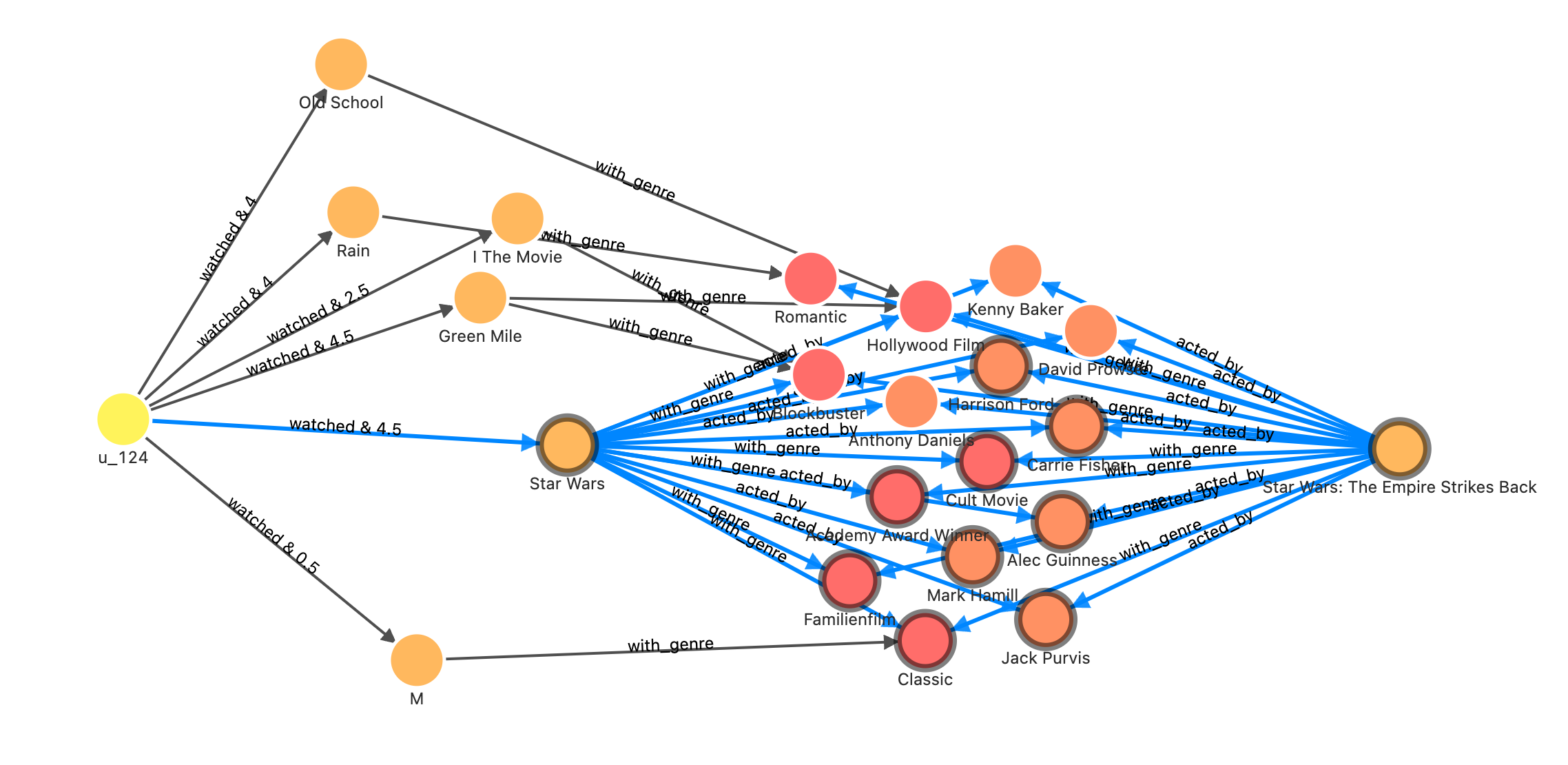# 「實操」結合圖資料庫、圖演算法、機器學習、GNN 實現一個推薦系統## 基本概念

• 資料、特徵的處理
• 從特徵出發，生成推薦列表
• 過濾、排序推薦列表

### 基於內容的過濾

• 清晰的可解釋性，無論是對使用者的畫像分析，還是對物品的運算本身天然帶來了排序、過濾的可解釋性；
• 使用者資料輸入的獨立性，對指定的待推薦使用者來說，只需要單獨分析他們的畫像和歷史評分就足夠了；
• 規避新物品冷啟動問題，對於新的新增的物品，即使沒有任何歷史的使用者評價，也可以做出推薦；

• 特徵提取難度，比如：照片、影片等非純文字資料，它們的特徵提取依賴領域專家知識。舉個例子，電影推薦系統需要抽出導演、電影分類等領域知識作為特徵；
• 難以打破舒適圈，發掘使用者的潛在新興趣點；
• 新使用者冷啟動資料缺失問題，新使用者個人資訊少難以形成使用者畫像，進而缺少做物品畫像、特種距離運算的輸入；

### 基於協同過濾

• 無需對非結構化物品進行特徵分析，因為協同過濾關注的是使用者和物品之間的協同互動，這繞過了對物品領域知識處理的需求；
• 對使用者的個性化定製程度更強、更細，基於行為的分析使得對使用者偏好的劃分本質上是連續的（相比來說，對使用者做畫像的方法則是離散的），這樣的推薦結果會更加“千人千面”。同時，也會蘊含內容過濾、有限的畫像角度之下的“驚喜”推薦。

• 有新使用者和新物件上的冷啟動問題，因為它們身上都缺少歷史喜好行為的資訊；

## 基於圖的個性推薦

### 建立圖譜

Schema 如下：

• 頂點：
• user(user_id)
• movie(name)
• person(name, birthdate)
• genre(name)
• 邊：
• watched(rate(double))
• with_genre
• directed_by
• acted_by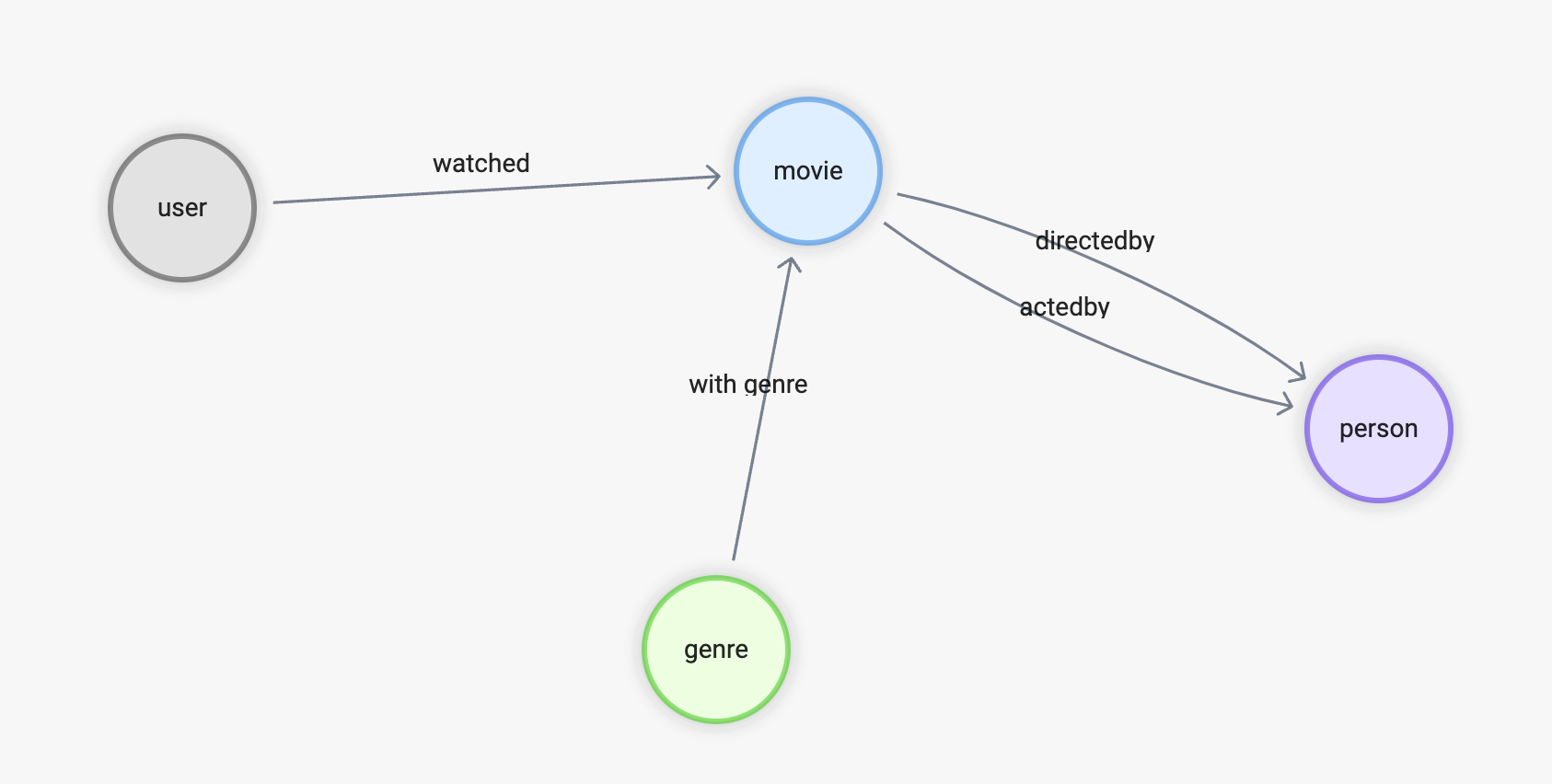1. 用 Nebula-Up 安裝 NebulaGraph；
2. 克隆 movie-recommendation-dataset；
3. 匯入資料集 NebulaGraph；
``````curl -fsSL nebula-up.siwei.io/install.sh | bash

git clone https://github.com/wey-gu/movie-recommendation-dataset.git && cd movie-recommendation-dataset

docker run --rm -ti \
--network=nebula-net \
-v \${PWD}:/root/ \
-v \${PWD}/dbt_project/to_nebulagraph/:/data \
vesoft/nebula-importer:v3.2.0 \
--config /root/nebula-importer.yaml
``````

### 基於內容的過濾

CBF，內容過濾的思想是利用領域知識、歷史記錄、元資料分別對使用者和物件做畫像、打標籤，最終根據使用者的標籤與待推薦物件之間的距離評分進行排序給出相關推薦。

CBF 的方法中沒有限定具體實現方式。如前邊介紹，可能是基於機器學習、Elasticsearch、圖譜等不同方法。為切合本章的主題，這裡我給出一個基於圖資料庫、圖譜上的 CBF 的例子，做一個電影推薦系統，能讓讀者理解這個方法的思想。同時，也能熟悉圖資料庫、知識圖譜的方法。

#### Jaccard Index

Jaccard Index 是一個描述兩個集合距離的定義公式，非常簡單、符合直覺地取兩者的交集與並集測度的比例，它的定義記為：#### CBF 方法在 NebulaGraph 中的實現

CBF 方法分如下四步：

1. 找出推薦使用者評分過的電影；
2. 從使用者評分過的電影，經由導演卡司、電影型別找到新的待推薦電影；
3. 對看過的電影與新的電影，藉由導演、卡司、電影型別的關係，在圖上做 Jaccard 相似性運算，得出每一對看過的電影和待推薦新電影之間的 Jaccard 係數；
4. 把使用者對看過電影的評分作為加權係數，針對其到每一個新電影之間的 Jaccard 係數加權評分，獲得排序後的推薦電影列表；
``````// 使用者 u_124 看過的電影
MATCH (u:`user`)-[:watched]->(m:`movie`) WHERE id(u) == "u_124"
WITH collect(id(m)) AS watched_movies_id

// 根據電影的標註關係找到備選推薦電影，刨除看過的，把評分、交集關聯鏈路的數量傳下去
MATCH (u:`user`)-[e:watched]->(m:`movie`)-[:directed_by|acted_by|with_genre]->(intersection)<-[:directed_by|acted_by|with_genre]-(recomm:`movie`)
WHERE id(u) == "u_124" AND NOT id(recomm) IN watched_movies_id
WITH (e.rate - 2.5)/2.5 AS rate, m, recomm, size(COLLECT(intersection)) AS intersection_size ORDER BY intersection_size DESC LIMIT 50

// 計算 Jaccard index-------------------------------------------------

// 針對每一對 m 和 recomm：
// 開始計算看過的電影，集合 a 的部分
MATCH (m:`movie`)-[:directed_by|acted_by|with_genre]->(intersection)
WITH rate, id(m) AS m_id, recomm, intersection_size, COLLECT(id(intersection)) AS set_a

// 計算推薦電影，集合 b 的部分
MATCH (recomm:`movie`)-[:directed_by|acted_by|with_genre]->(intersection)
WITH rate, m_id, id(recomm) AS recomm_id, set_a, intersection_size, COLLECT(id(intersection)) AS set_b

// 得到並集數量
WITH rate, m_id, recomm_id, toFloat(intersection_size) AS intersection_size, toSet(set_a + set_b) AS A_U_B

// 得到每一對 m 和 recomm 的 Jaccard index = A_N_B/A_U_B
WITH rate, m_id, recomm_id, intersection_size/size(A_U_B) AS jaccard_index

// 計算 Jaccard index-------------------------------------------------

// 得到每一個被推薦的電影 recomm_id，經由不同看過電影推薦鏈路的相似度 = 評分 * jaccard_index
WITH recomm_id, m_id, (rate * jaccard_index) AS score

// 對每一個 recomm_id 按照 m_id 加權求得相似度的和，為總的推薦程度評分，降序排列
WITH recomm_id, sum(score) AS sim_score ORDER BY sim_score DESC WHERE sim_score > 0
RETURN recomm_id, sim_score LIMIT 50
``````

recomm_id sim_score
1891 0.2705882352941177
1892 0.22278481012658227
1894 0.15555555555555556
808 0.144
1895 0.13999999999999999
85 0.12631578947368421
348 0.12413793103448277
18746 0.11666666666666668
628 0.11636363636363636
3005 0.10566037735849057

#### 視覺化分析

``````// 使用者 u_124 看過的電影
MATCH p=(u:`user`)-[:watched]->(m:`movie`) WHERE id(u) == "u_124"
RETURN p
``````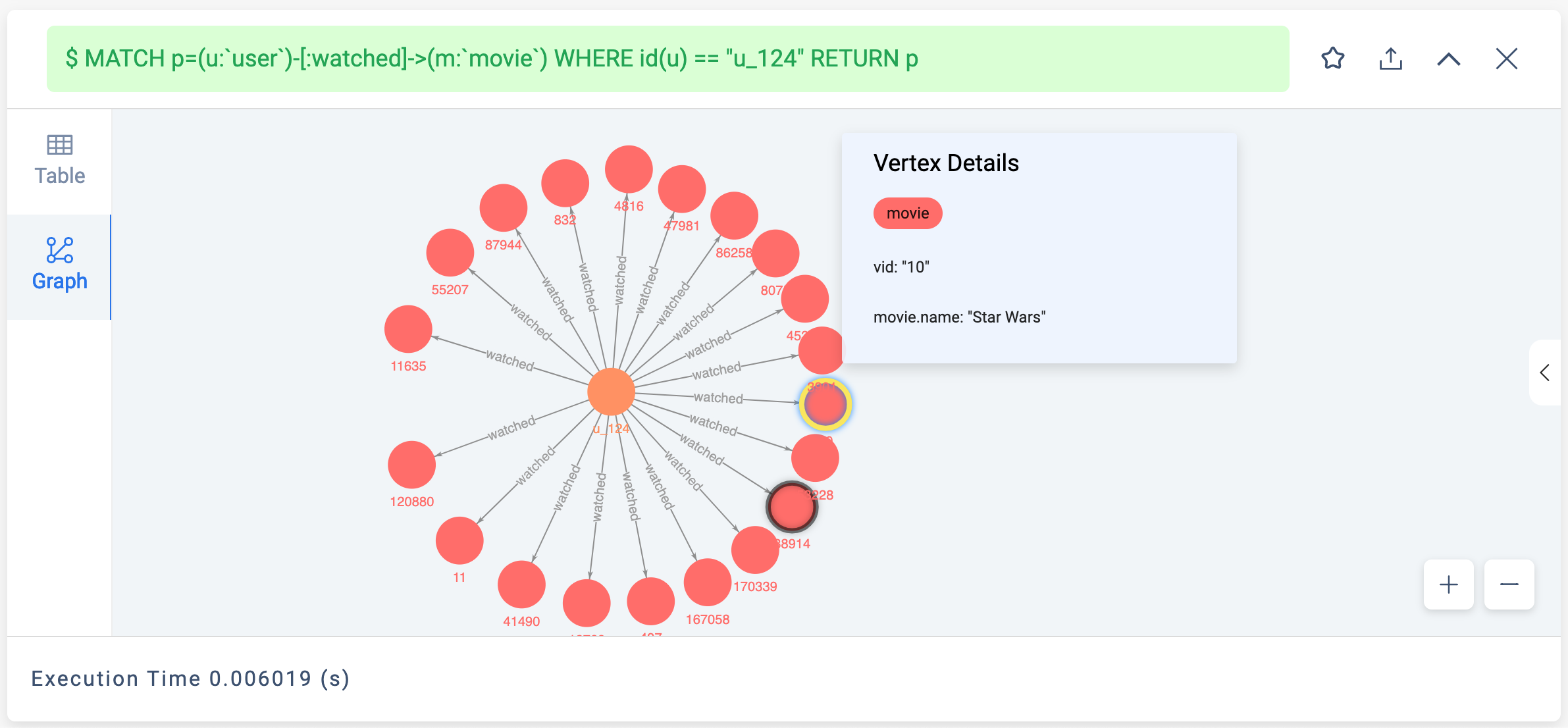``````// 使用者 u_124 看過的電影
MATCH (u:`user`)-[:watched]->(m:`movie`) WHERE id(u) == "u_124"
WITH collect(id(m)) AS watched_movies_id

// 根據電影的標註關係找到備選推薦電影，刨除看過的，把評分、交集關聯鏈路的數量傳下去
MATCH p=(u:`user`)-[e:watched]->(m:`movie`)-[:directed_by|acted_by|with_genre]->(intersection)<-[:directed_by|acted_by|with_genre]-(recomm:`movie`)
WHERE id(u) == "u_124" AND NOT id(recomm) IN watched_movies_id
RETURN p, size(COLLECT(intersection)) AS intersection_size ORDER BY intersection_size DESC LIMIT 500
``````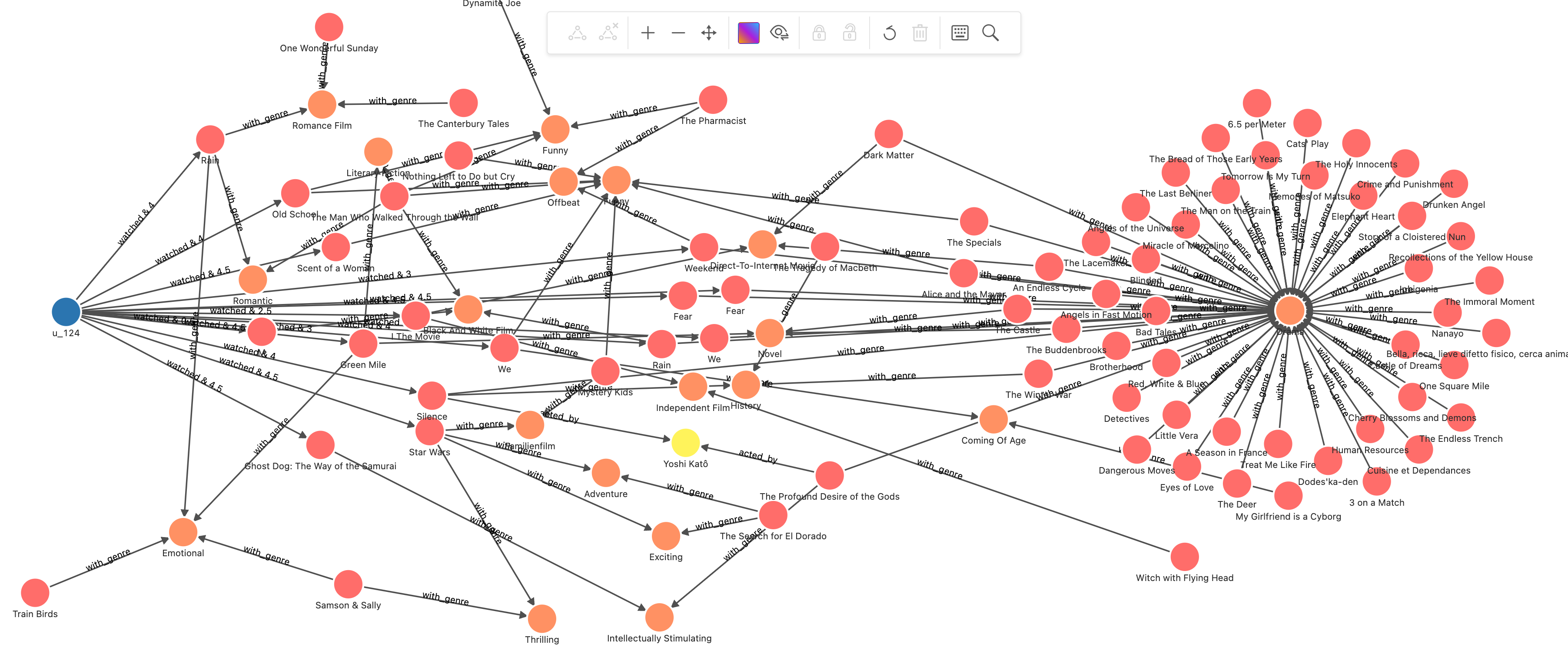``````// 使用者 u_124 看過的電影
MATCH (u:`user`)-[:watched]->(m:`movie`) WHERE id(u) == "u_124"
WITH collect(id(m)) AS watched_movies_id

// 根據電影的標註關係找到備選推薦電影，刨除看過的，把評分、交集關聯鏈路的數量傳下去
MATCH (u:`user`)-[e:watched]->(m:`movie`)-[:directed_by|acted_by|with_genre]->(intersection)<-[:directed_by|acted_by|with_genre]-(recomm:`movie`)
WHERE id(u) == "u_124" AND NOT id(recomm) IN watched_movies_id
WITH (e.rate - 2.5)/2.5 AS rate, m, recomm, size(COLLECT(intersection)) AS intersection_size ORDER BY intersection_size DESC LIMIT 50

// 計算 Jaccard index-------------------------------------------------

// 針對每一對 m 和 recomm：
// 開始計算看過的電影，集合 a 的部分
MATCH (m:`movie`)-[:directed_by|acted_by|with_genre]->(intersection)
WITH rate, id(m) AS m_id, recomm, intersection_size, COLLECT(id(intersection)) AS set_a

// 計算推薦電影，集合 b 的部分
MATCH (recomm:`movie`)-[:directed_by|acted_by|with_genre]->(intersection)
WITH rate, m_id, id(recomm) AS recomm_id, set_a, intersection_size, COLLECT(id(intersection)) AS set_b

// 得到並集數量
WITH rate, m_id, recomm_id, toFloat(intersection_size) AS intersection_size, toSet(set_a + set_b) AS A_U_B

// 得到每一對 m 和 recomm 的 Jaccard index = A_N_B/A_U_B
WITH rate, m_id, recomm_id, intersection_size/size(A_U_B) AS jaccard_index

// 計算 Jaccard index-------------------------------------------------

// 得到每一個被推薦的電影 recomm_id，經由不同看過電影推薦鏈路的相似度 = 評分 * jaccard_index
WITH recomm_id, m_id, (rate * jaccard_index) AS score

// 對每一個 recomm_id 按照 m_id 加權求得相似度的和，為總的推薦程度評分，降序排列
WITH recomm_id, sum(score) AS sim_score ORDER BY sim_score DESC WHERE sim_score > 0
WITH recomm_id, sim_score LIMIT 10
WITH COLLECT(recomm_id) AS recomm_ids
MATCH p = (u:`user`)-[e:watched]->(m:`movie`)-[:directed_by|acted_by|with_genre]->(intersection)<-[:directed_by|acted_by|with_genre]-(recomm:`movie`)
WHERE id(u) == "u_124" AND id(recomm) in recomm_ids
RETURN p
``````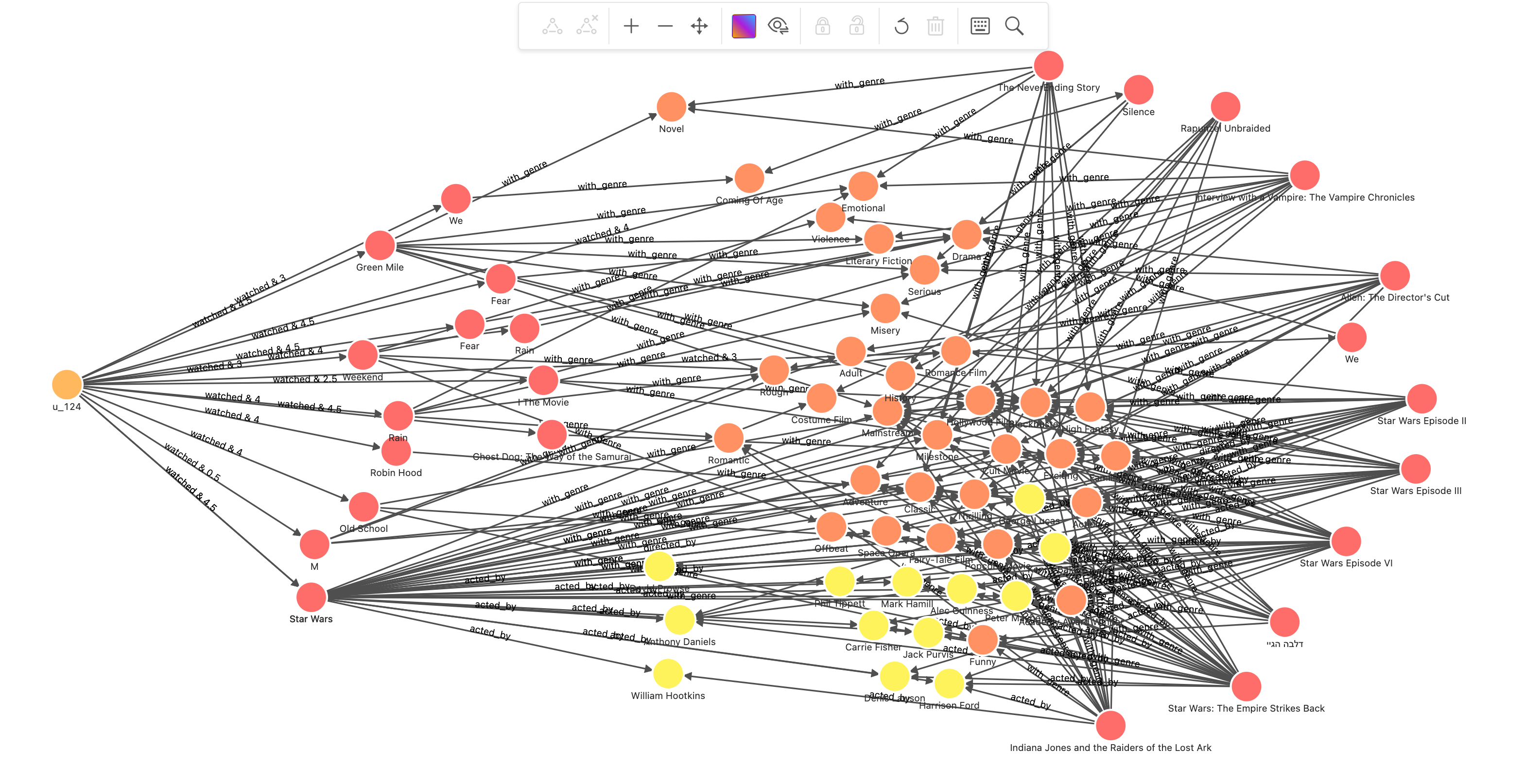### 基於記憶的協同過濾

• User-User CF 基於多個使用者對物件的歷史行為，判定使用者之間的相似性，再根據相似使用者的選擇推薦新的物件；
• Item-Item CF 判斷物件之間的相似性，給使用者推薦他喜歡的物品相似的物品。

#### ItemCF

1. 找出推薦使用者評分過的電影；
2. 經由使用者的高評分電影，找到其他給出高評分使用者所看過的新的高評分電影；
3. 通過使用者的評分對看過的電影與新的電影在圖上做 Jaccard 相似性運算，得出每一對看過的電影和待推薦新電影之間的 Jaccard 係數；
4. 把使用者對看過電影的評分作為加權係數，針對其到每一個新電影之間的 Jaccard 係數加權評分，獲得排序後的推薦電影列表。
``````// 使用者 u_124 看過的並給出高評分的電影
MATCH (u:`user`)-[e:watched]->(m:`movie`) WHERE id(u) == "u_124" AND e.rate > 3
WITH collect(id(m)) AS watched_movies_id

// 根據同樣也看過這些電影，並給出高評分的使用者，得出待推薦的電影
MATCH (u:`user`)-[e:watched]->(m:`movie`)<-[e0:watched]-(intersection:`user`)-[e1:watched]->(recomm:`movie`)
WHERE id(u) == "u_124" AND NOT id(recomm) IN watched_movies_id AND e0.rate >3 AND e1.rate > 3
WITH (e.rate - 2.5)/2.5 AS rate, m, recomm, size(COLLECT(intersection)) AS intersection_size ORDER BY intersection_size DESC LIMIT 50

// 計算 Jaccard index-------------------------------------------------

// 針對每一對 m 和 recomm：
// 開始計算看過的電影，集合 a 的部分
MATCH (m:`movie`)<-[e0:watched]-(intersection:`user`)
WHERE e0.rate >3
WITH rate, id(m) AS m_id, recomm, intersection_size, COLLECT(id(intersection)) AS set_a

// 計算推薦電影，集合 b 的部分
MATCH (recomm:`movie`)<-[e1:watched]-(intersection:`user`)
WHERE e1.rate >3
WITH rate, m_id, id(recomm) AS recomm_id, set_a, intersection_size, COLLECT(id(intersection)) AS set_b

// 得到並集數量
WITH rate, m_id, recomm_id, toFloat(intersection_size) AS intersection_size, toSet(set_a + set_b) AS A_U_B

// 得到每一對 m 和 recomm 的 Jaccard index = A_N_B/A_U_B
WITH rate, m_id, recomm_id, intersection_size/size(A_U_B) AS jaccard_index

// 計算 Jaccard index-------------------------------------------------

// 得到每一個被推薦的電影 recomm_id，經由不同看過電影推薦鏈路的相似度 = 評分 * jaccard_index
WITH recomm_id, m_id, (rate * jaccard_index) AS score

// 對每一個 recomm_id 按照 m_id 加權求得相似度的和，為總的推薦程度評分，降序排列，只取正值
WITH recomm_id, sum(score) AS sim_score ORDER BY sim_score DESC WHERE sim_score > 0
RETURN recomm_id, sim_score LIMIT 50
``````

recomm_id sim_score
832 0.8428369424692955
114707 0.7913842214590154
64957 0.6924673321504288
120880 0.5775219768736295
807 0.497532028328161
473 0.4748322300870322
52797 0.2311965559170528
12768 0.19642857142857142
167058 0.19642857142857142

#### 視覺化分析 ItemCF

``````// 使用者 u_124 看過的並給出高評分的電影
MATCH p=(u:`user`)-[e:watched]->(m:`movie`) WHERE id(u) == "u_124" AND e.rate > 3
RETURN p
``````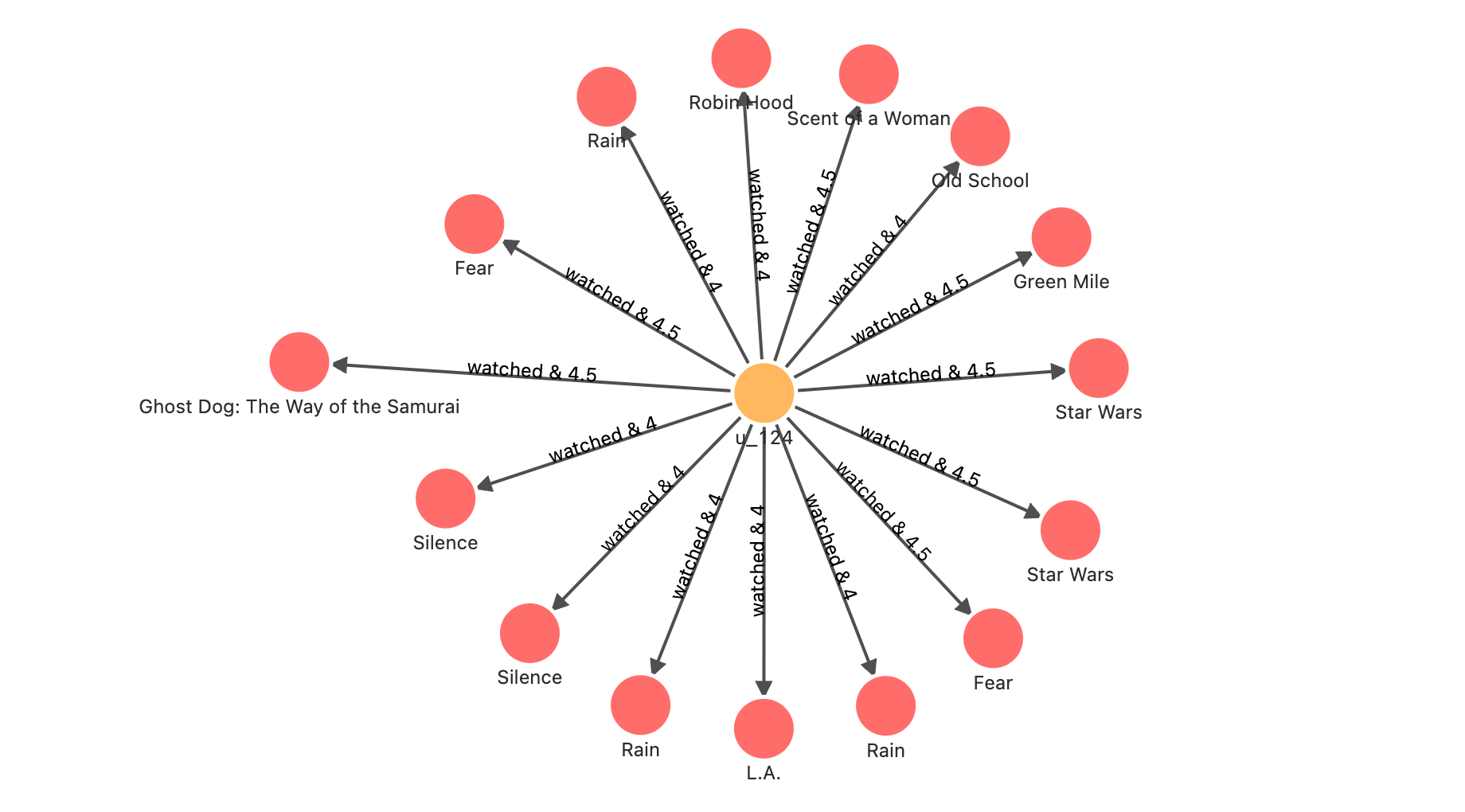``````// 使用者 u_124 看過的並給出高評分的電影
MATCH (u:`user`)-[e:watched]->(m:`movie`) WHERE id(u) == "u_124" AND e.rate > 3
WITH collect(id(m)) AS watched_movies_id

// 根據同樣也看過這些電影，並給出高評分的使用者，得出待推薦的電影
MATCH p=(u:`user`)-[e:watched]->(m:`movie`)<-[e0:watched]-(intersection:`user`)-[e1:watched]->(recomm:`movie`)
WHERE id(u) == "u_124" AND NOT id(recomm) IN watched_movies_id AND e0.rate >3 AND e1.rate > 3
WITH p, size(COLLECT(intersection)) AS intersection_size ORDER BY intersection_size DESC LIMIT 200
``````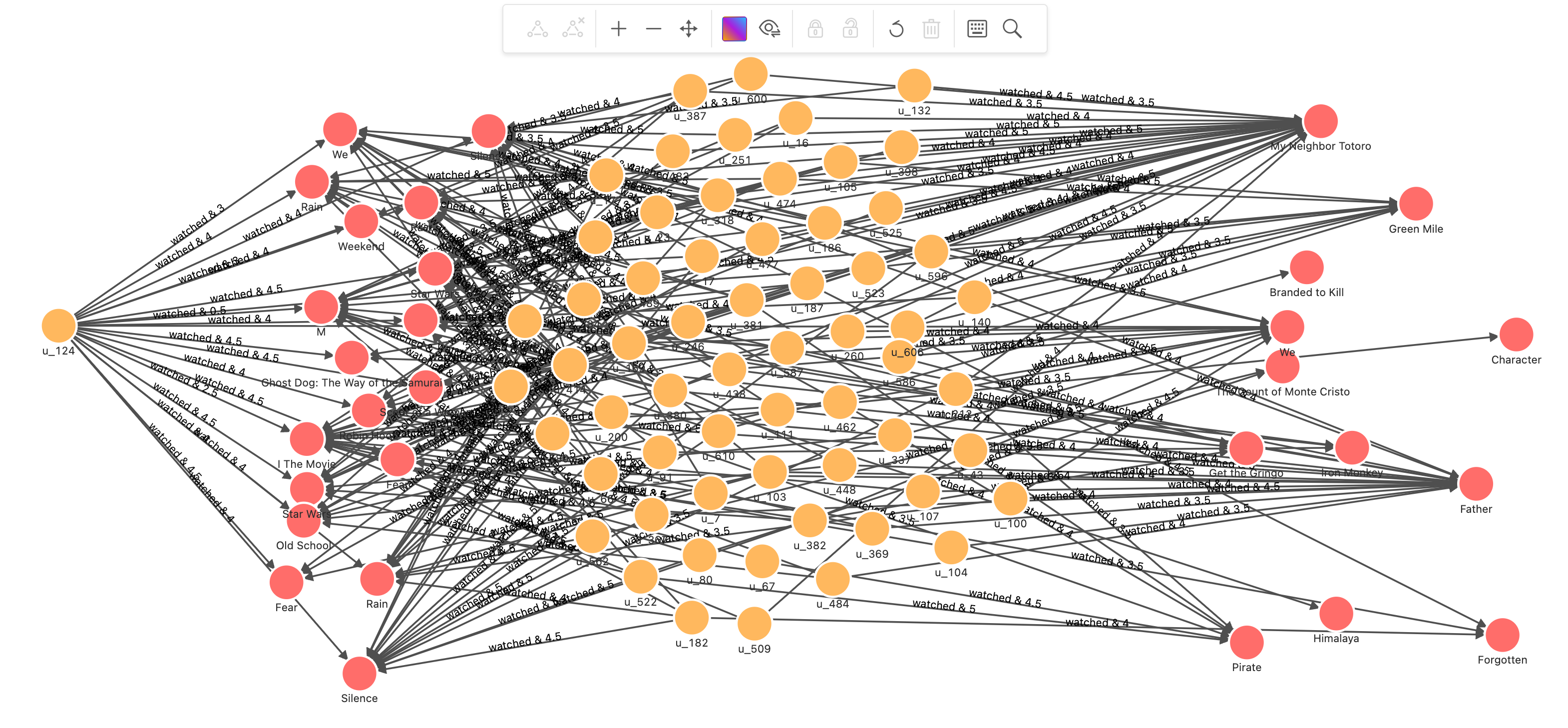``````// 使用者 u_124 看過的並給出高評分的電影
MATCH (u:`user`)-[e:watched]->(m:`movie`) WHERE id(u) == "u_124" AND e.rate > 3
WITH collect(id(m)) AS watched_movies_id

// 根據同樣也看過這些電影，並給出高評分的使用者，得出待推薦的電影
MATCH (u:`user`)-[e:watched]->(m:`movie`)<-[e0:watched]-(intersection:`user`)-[e1:watched]->(recomm:`movie`)
WHERE id(u) == "u_124" AND NOT id(recomm) IN watched_movies_id AND e0.rate >3 AND e1.rate > 3
WITH (e.rate - 2.5)/2.5 AS rate, m, recomm, size(COLLECT(intersection)) AS intersection_size ORDER BY intersection_size DESC LIMIT 50

// 計算 Jaccard index-------------------------------------------------

// 針對每一對 m 和 recomm：
// 開始計算看過的電影，集合 a 的部分
MATCH (m:`movie`)<-[e0:watched]-(intersection:`user`)
WHERE e0.rate >3
WITH rate, id(m) AS m_id, recomm, intersection_size, COLLECT(id(intersection)) AS set_a

// 計算推薦電影，集合 b 的部分
MATCH (recomm:`movie`)<-[e1:watched]-(intersection:`user`)
WHERE e1.rate >3
WITH rate, m_id, id(recomm) AS recomm_id, set_a, intersection_size, COLLECT(id(intersection)) AS set_b

// 得到並集數量
WITH rate, m_id, recomm_id, toFloat(intersection_size) AS intersection_size, toSet(set_a + set_b) AS A_U_B

// 得到每一對 m 和 recomm 的 Jaccard index = A_N_B/A_U_B
WITH rate, m_id, recomm_id, intersection_size/size(A_U_B) AS jaccard_index

// 計算 Jaccard index-------------------------------------------------

// 得到每一個被推薦的電影 recomm_id，經由不同看過電影推薦鏈路的相似度 = 評分 * jaccard_index
WITH recomm_id, m_id, (rate * jaccard_index) AS score

// 對每一個 recomm_id 按照 m_id 加權求得相似度的和，為總的推薦程度評分，降序排列，只取正值
WITH recomm_id, sum(score) AS sim_score ORDER BY sim_score DESC WHERE sim_score > 0
WITH recomm_id LIMIT 10
WITH COLLECT(recomm_id) AS recomm_ids
MATCH p = (u:`user`)-[e:watched]->(m:`movie`)<-[e0:watched]-(intersection:`user`)-[e1:watched]->(recomm:`movie`)
WHERE id(u) == "u_124" AND id(recomm) in recomm_ids
RETURN p limit 500
``````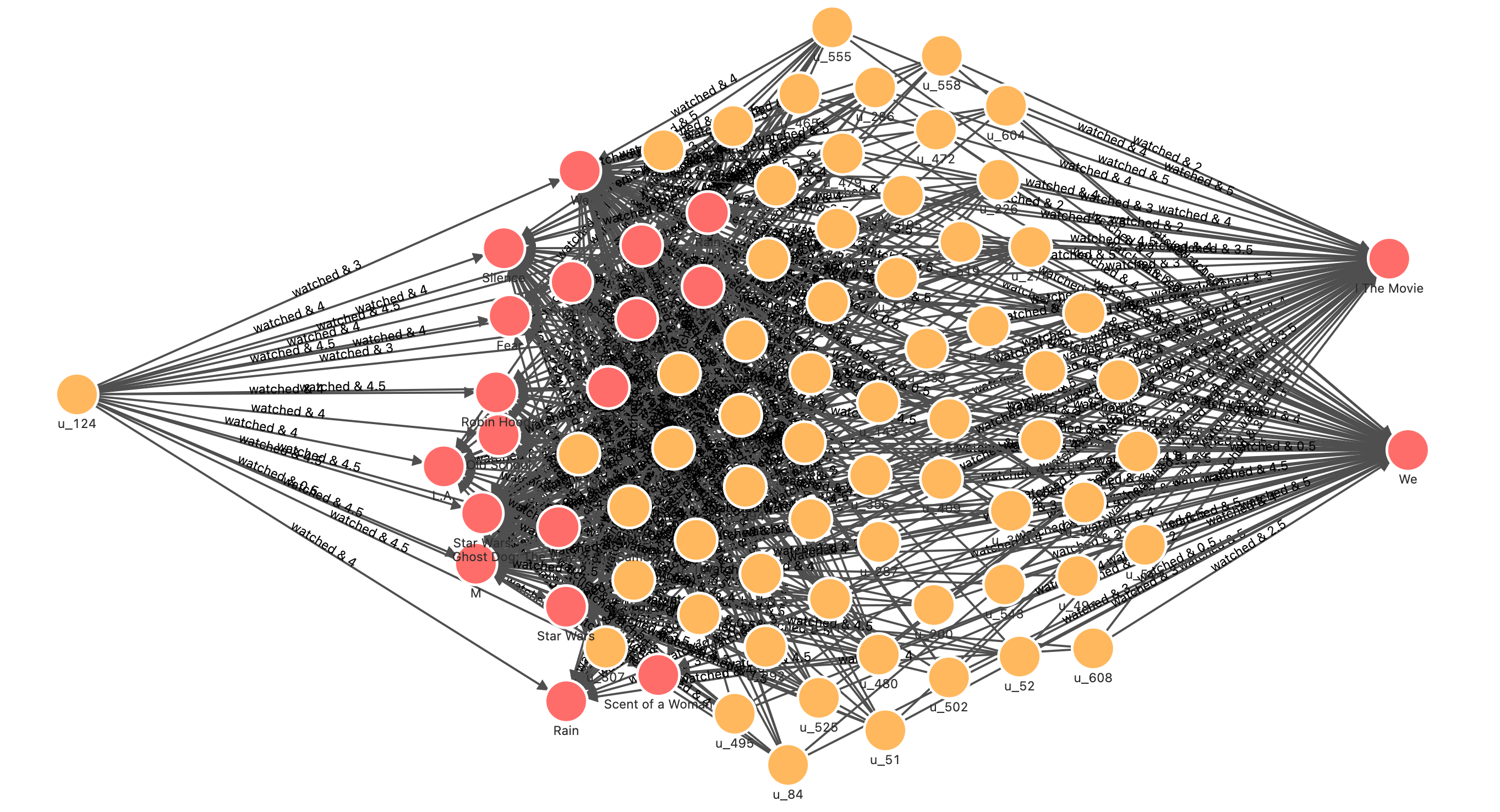#### 關於”高評分“

``````(m:`movie`)<-[e0:watched]-(intersection:`user`)-[e1:watched]->(recomm:`movie`)
``````

e0 與 e1 的評分數值的作用因素只過濾了低評分的關係，可以做進一步優化。

#### Pearson Correlation Coefficient

Pearson Correlation Coefficient，縮寫 PCC。其定義為：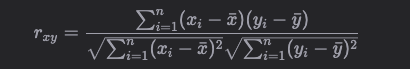#### UserCF

1. 找出和推薦使用者同樣給出評分過的電影的使用者；
2. 運算 Pearson Correlation Coefficient 得到和推薦使用者興趣接近的使用者；
3. 通過興趣接近使用者得到高評分未觀看電影；
4. 根據觀看使用者的 Pearson Correlation Coefficient 加權，排序得推薦電影列表；
``````// 找出和使用者 u_2 看過相同電影的使用者, 得電影評分
MATCH (u:`user`)-[e0:watched]->(m:`movie`)<-[e1:watched]-(u_sim:`user`)
WHERE id(u) == "u_2" AND id(u_sim) != "u_2"
WITH u, u_sim, collect(id(m)) AS watched_movies_id, COLLECT({e0: e0, e1: e1}) AS e

// 計算 u_2 和這些使用者的 pearson_cc
MATCH (u:`user`)-[e0:watched]->(m:`movie`)
WITH u_sim, watched_movies_id, avg(e0.rate) AS u_mean, e

MATCH (u_sim:`user`)-[e1:watched]->(recomm:`movie`)
WITH u_sim, watched_movies_id, u_mean, avg(e1.rate) AS u_sim_mean, e AS e_

UNWIND e_ AS e
WITH sum((e.e0.rate - u_mean) * (e.e1.rate - u_sim_mean) ) AS numerator,
sqrt(sum(exp2(e.e0.rate - u_mean)) * sum(exp2(e.e1.rate - u_sim_mean))) AS denominator,
u_sim, watched_movies_id WHERE denominator != 0

// 取 pearson_cc 最大的 50 個相似使用者
WITH u_sim, watched_movies_id, numerator/denominator AS pearson_cc ORDER BY pearson_cc DESC LIMIT 50

// 取相似使用者給出高評分的新電影，根據相似使用者個數對使用者相似程度 pearson_cc 加權，獲得推薦列表
MATCH (u_sim:`user`)-[e1:watched]->(recomm:`movie`)
WHERE NOT id(recomm) IN watched_movies_id AND e1.rate > 3
RETURN recomm, sum(pearson_cc) AS sim_score ORDER BY sim_score DESC LIMIT 50
``````

recomm sim_score
("120880" :movie{name: "I The Movie"}) 33.018868012270396
("167058" :movie{name: "We"}) 22.38531462958867
("12768" :movie{name: "We"}) 22.38531462958867
("55207" :movie{name: "Silence"}) 22.342886447570585
("170339" :movie{name: "Silence"}) 22.342886447570585
("114707" :movie{name: "Raid"}) 21.384280909249796
("10" :movie{name: "Star Wars"}) 19.51546960750133
("11" :movie{name: "Star Wars"}) 19.515469607501327
("64957" :movie{name: "Mat"}) 18.142639694676603
("187689" :movie{name: "Sin"}) 18.078111338733557

#### 視覺化分析 UserCF

``````// 找出和使用者 u_2 看過相同電影的使用者
MATCH p=(u:`user`)-[e0:watched]->(m:`movie`)<-[e1:watched]-(u_sim:`user`)
WHERE id(u) == "u_2" AND id(u_sim) != "u_2"
RETURN p
``````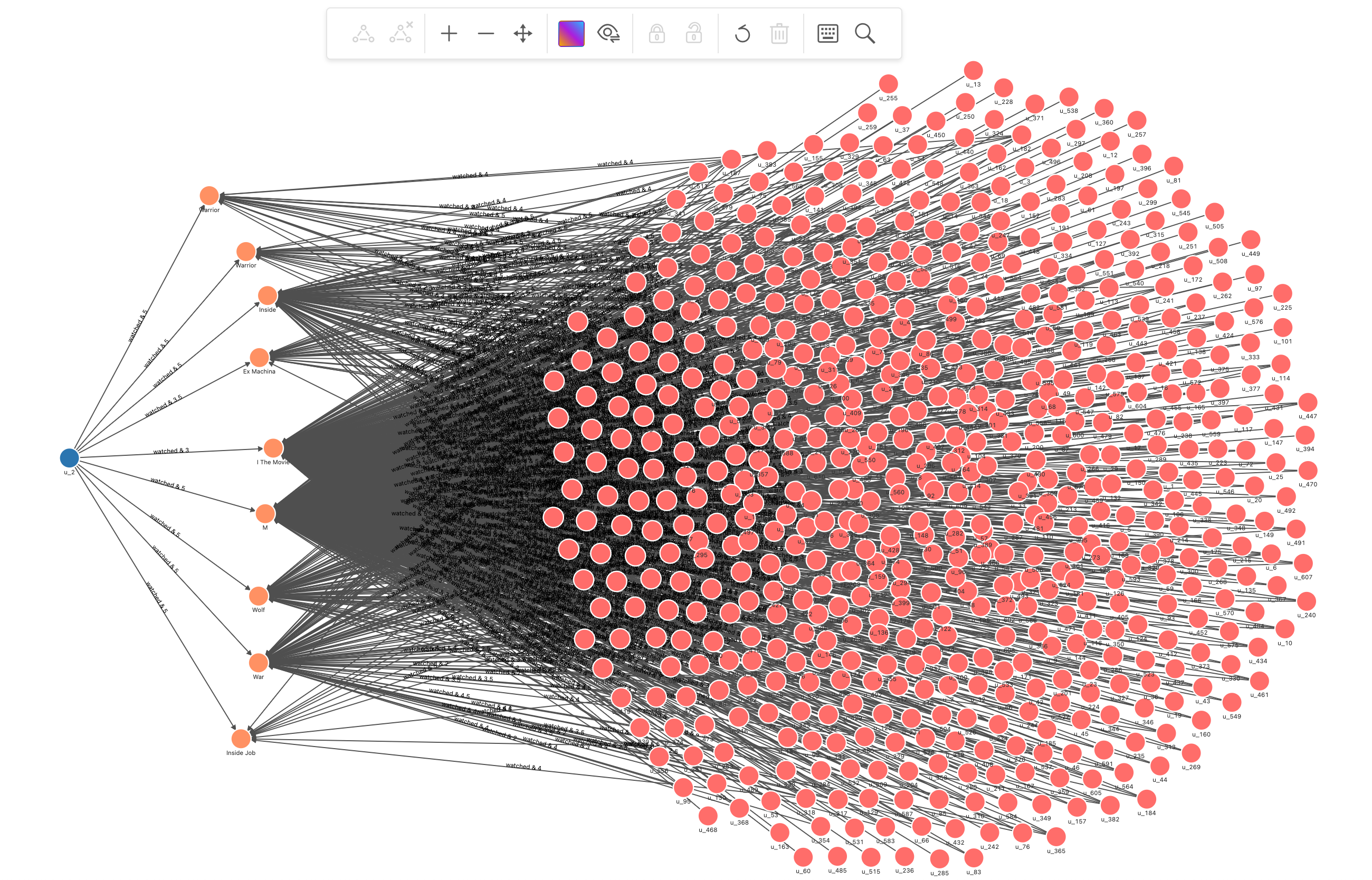``````// 找出和使用者 u_2 看過相同電影的使用者, 得電影評分
MATCH (u:`user`)-[e0:watched]->(m:`movie`)<-[e1:watched]-(u_sim:`user`)
WHERE id(u) == "u_2" AND id(u_sim) != "u_2"
WITH u, u_sim, collect(id(m)) AS watched_movies_id, COLLECT({e0: e0, e1: e1}) AS e

// 計算 u_2 和這些使用者的 pearson_cc
MATCH (u:`user`)-[e0:watched]->(m:`movie`)
WITH u_sim, watched_movies_id, avg(e0.rate) AS u_mean, e

MATCH (u_sim:`user`)-[e1:watched]->(recomm:`movie`)
WITH u_sim, watched_movies_id, u_mean, avg(e1.rate) AS u_sim_mean, e AS e_

UNWIND e_ AS e
WITH sum((e.e0.rate - u_mean) * (e.e1.rate - u_sim_mean) ) AS numerator,
sqrt(sum(exp2(e.e0.rate - u_mean)) * sum(exp2(e.e1.rate - u_sim_mean))) AS denominator,
u_sim, watched_movies_id WHERE denominator != 0

// 取 pearson_cc 最大的 50 個相似使用者
WITH u_sim, watched_movies_id, numerator/denominator AS pearson_cc ORDER BY pearson_cc DESC LIMIT 50

RETURN u_sim
``````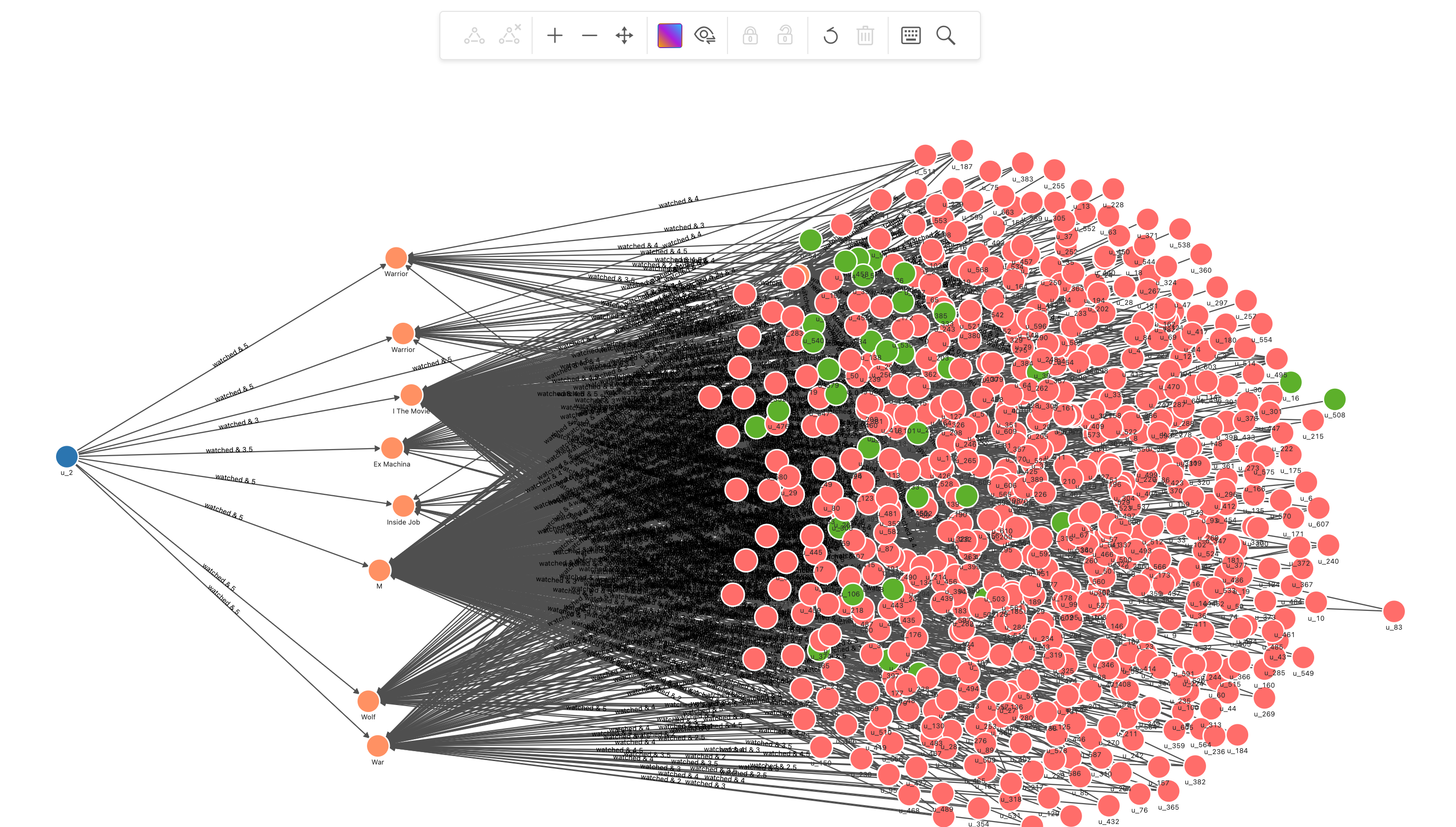``````// 找出和使用者 u_2 看過相同電影的使用者, 得電影評分
MATCH (u:`user`)-[e0:watched]->(m:`movie`)<-[e1:watched]-(u_sim:`user`)
WHERE id(u) == "u_2" AND id(u_sim) != "u_2"
WITH u, u_sim, collect(id(m)) AS watched_movies_id, COLLECT({e0: e0, e1: e1}) AS e

// 計算 u_2 和這些使用者的 pearson_cc
MATCH (u:`user`)-[e0:watched]->(m:`movie`)
WITH u_sim, watched_movies_id, avg(e0.rate) AS u_mean, e

MATCH (u_sim:`user`)-[e1:watched]->(recomm:`movie`)
WITH u_sim, watched_movies_id, u_mean, avg(e1.rate) AS u_sim_mean, e AS e_

UNWIND e_ AS e
WITH sum((e.e0.rate - u_mean) * (e.e1.rate - u_sim_mean) ) AS numerator,
sqrt(sum(exp2(e.e0.rate - u_mean)) * sum(exp2(e.e1.rate - u_sim_mean))) AS denominator,
u_sim, watched_movies_id WHERE denominator != 0

// 取 pearson_cc 最大的 50 個相似使用者
WITH u_sim, watched_movies_id, numerator/denominator AS pearson_cc ORDER BY pearson_cc DESC LIMIT 50

// 取相似使用者給出高評分的新電影，根據相似使用者個數對使用者相似程度 pearson_cc 加權，獲得推薦列表
MATCH p=(u_sim:`user`)-[e1:watched]->(recomm:`movie`)
WHERE NOT id(recomm) IN watched_movies_id AND e1.rate > 3
WITH p, recomm, sum(pearson_cc) AS sim_score ORDER BY sim_score DESC LIMIT 50
RETURN p
``````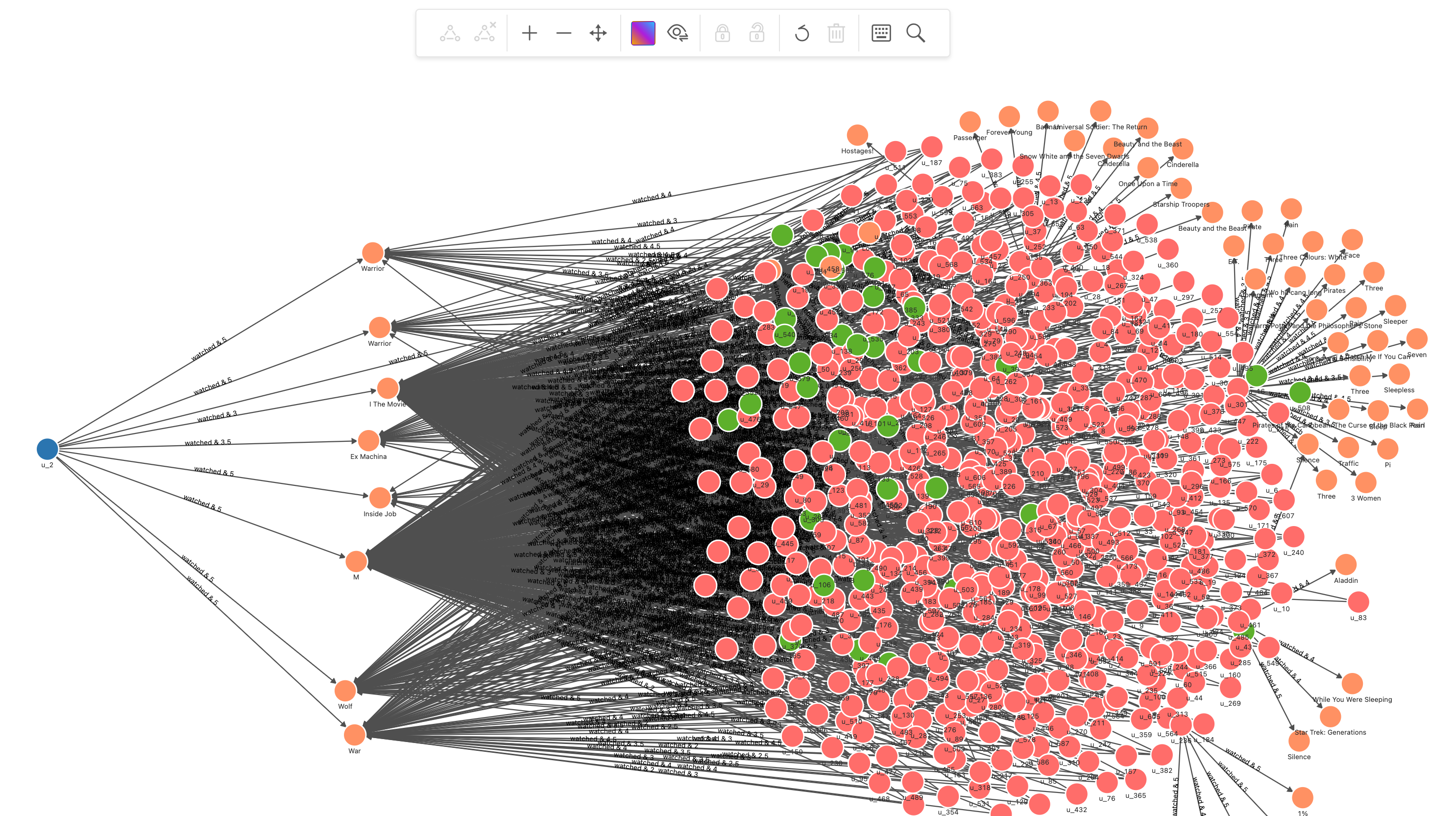### 混合的方法

#### 基於模型的方法

GNN 的方法可以將圖譜中的內容資訊（導演、演員、型別）和協同資訊（使用者-使用者、電影-電影、使用者-電影之間的相互關係）以知識的方式嵌入，並且方法中的訊息傳遞方式保有了圖中的區域性性（locality），這使得它可能成為一個非常新穎、有效的推薦系統模型方法。

#### GNN + 圖資料庫的推薦系統

GNN 的方法中，圖資料庫只是一個可選項，而我給的 GNN 方法的示例中，圖資料庫的關鍵作用是它帶來了實時性的可能

• 輸入資料可以實時、近實時獲取；
• 推理運算可以實時完成；

• 左邊是模型訓練，在圖譜的二分圖（user 和 item）之上建模
• 使用者和物件（movie）之間的關係除了互動關係之外，還有預先處理的關係，這些關係被查詢獲得後再寫回圖資料庫中供後續消費使用，關係有：
• 使用者間“相似”關係
• 物件間不同（共同演員、型別、導演）關係
• 利用 Nebula-DGL 將圖中需要的點、邊序列化為圖深度學習框架 DGL 可以消費的物件
• 在 DGL 中分割訓練、測試、驗證集，利用 PinSAGE 模型訓練
• 匯出模型給推薦系統使用
• 右邊是匯出的模型作為推理介面的推薦系統
• 基於相簿的實時圖譜上一直會有實時的資料更新，節點增減
• 當給定的使用者推薦請求過來的時候，相簿中以該使用者為起點的子圖會被獲取（1.）、作為輸入傳送給推理介面（2.）
• 推理介面把子圖輸入給之前訓練的模型，獲得該使用者在子圖中關聯的新物件中的評分排序（3.）作為推薦結果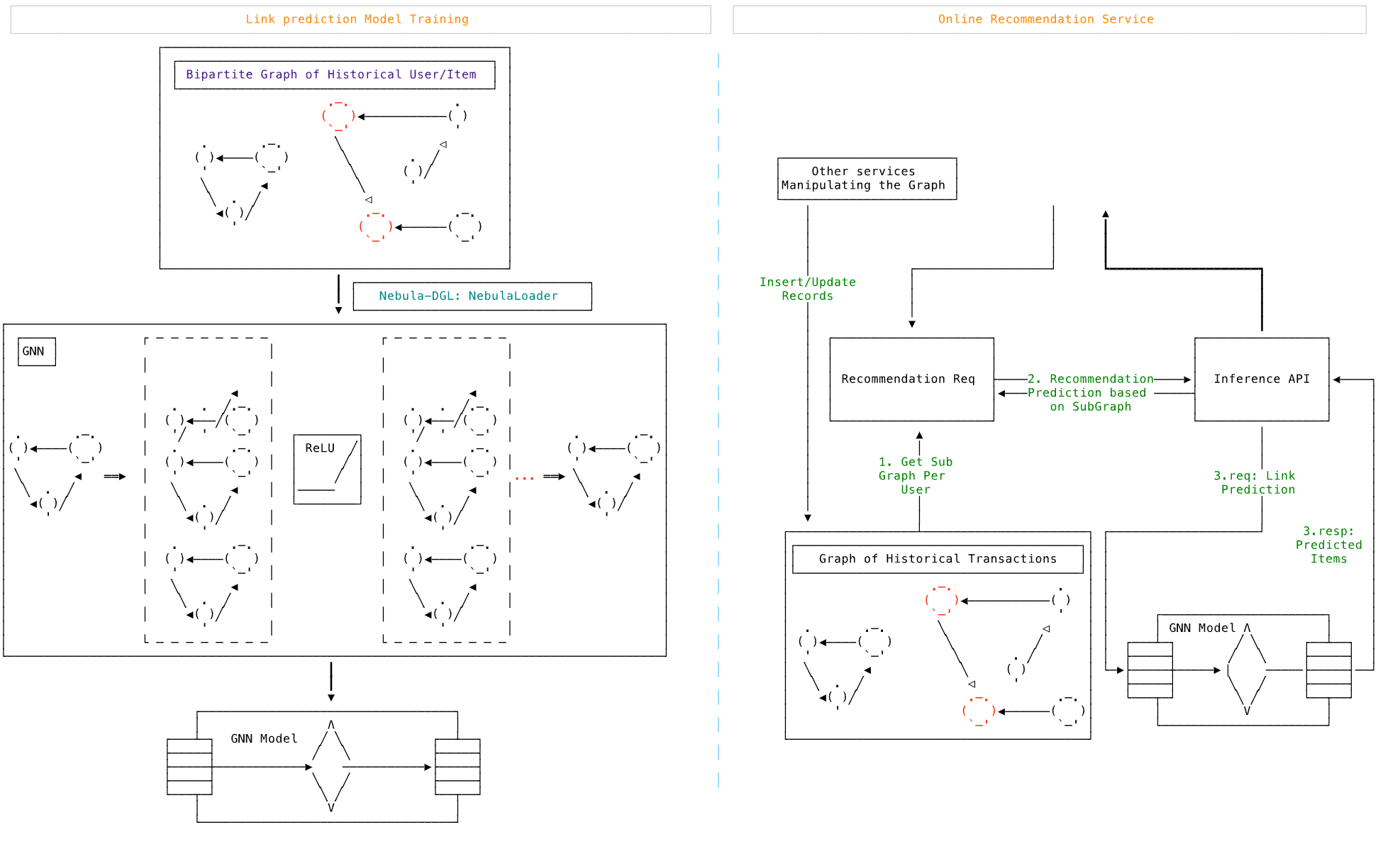## 推薦系統可解釋性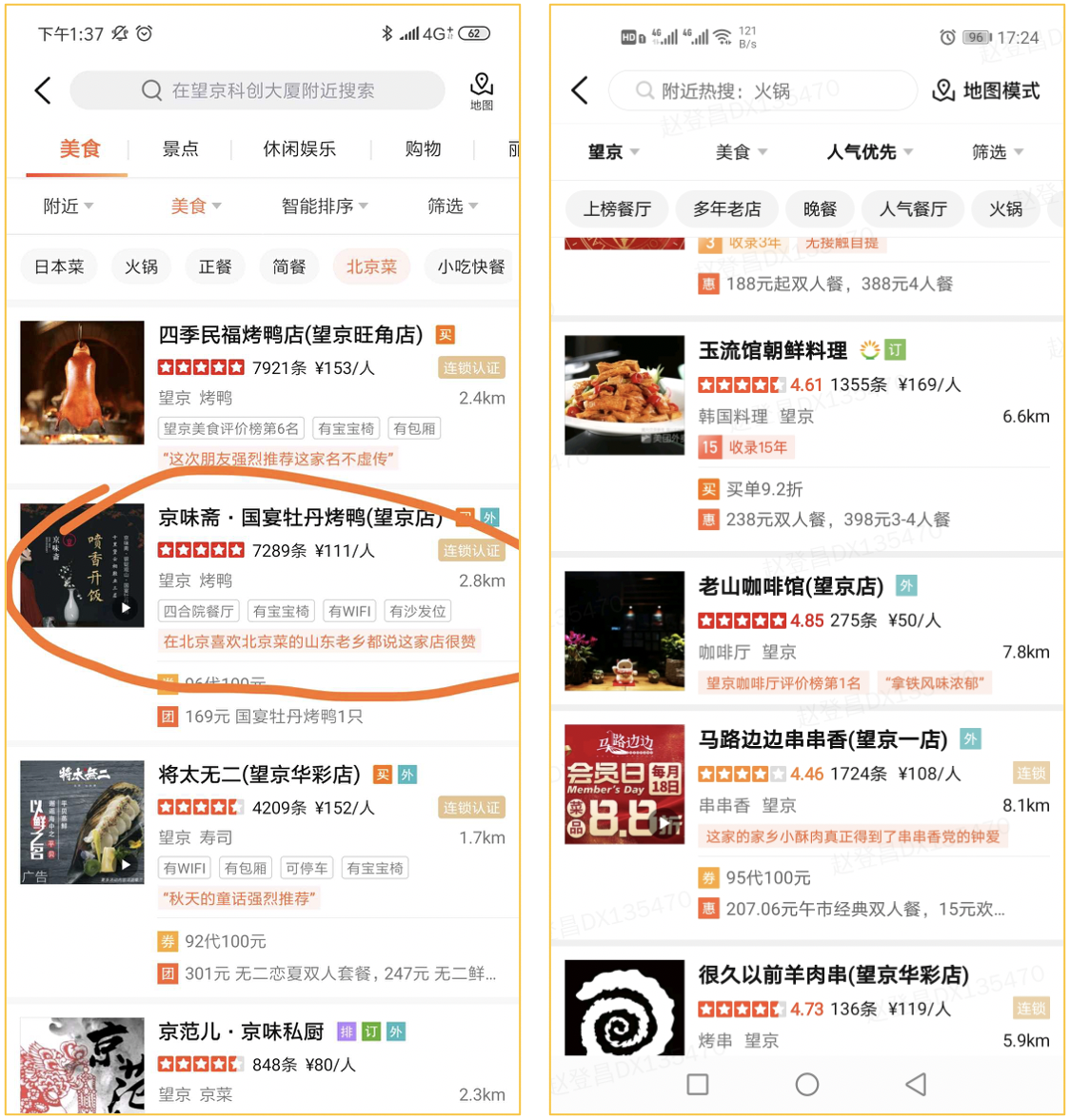#### 可解釋性的例子

``````FIND NOLOOP PATH FROM "u_124" TO "1891" over * BIDIRECT UPTO 4 STEPS yield path as `p` | LIMIT 20
``````

``````([email protected]) [moviegraph]> FIND NOLOOP PATH FROM "u_124" TO "1891" over * BIDIRECT UPTO 4 STEPS yield path as `p` | LIMIT 20
+-----------------------------------------------------------------------------------------------------+
| p                                                                                                   |
+-----------------------------------------------------------------------------------------------------+
| <("u_124")-[:[email protected] {}]->("11")-[:[email protected] {}]->("g_49")<-[:[email protected] {}]-("1891")>       |
| <("u_124")-[:[email protected] {}]->("11")-[:[email protected] {}]->("g_17")<-[:[email protected] {}]-("1891")>       |
| <("u_124")-[:[email protected] {}]->("11")-[:[email protected] {}]->("g_10281")<-[:[email protected] {}]-("1891")>    |
| <("u_124")-[:[email protected] {}]->("11")-[:[email protected] {}]->("p_4")<-[:[email protected] {}]-("1891")>            |
| <("u_124")-[:[email protected] {}]->("11")-[:[email protected] {}]->("p_3")<-[:[email protected] {}]-("1891")>            |
| <("u_124")-[:[email protected] {}]->("11")-[:[email protected] {}]->("p_24342")<-[:[email protected] {}]-("1891")>        |
| <("u_124")-[:[email protected] {}]->("11")-[:[email protected] {}]->("p_2")<-[:[email protected] {}]-("1891")>            |
| <("u_124")-[:[email protected] {}]->("832")-[:[email protected] {}]->("g_1110")<-[:[email protected] {}]-("1891")>    |
| <("u_124")-[:[email protected] {}]->("11")-[:[email protected] {}]->("g_1110")<-[:[email protected] {}]-("1891")>     |
| <("u_124")-[:[email protected] {}]->("11")-[:[email protected] {}]->("p_13463")<-[:[email protected] {}]-("1891")>        |
| <("u_124")-[:[email protected] {}]->("11")-[:[email protected] {}]->("p_12248")<-[:[email protected] {}]-("1891")>        |
| <("u_124")-[:[email protected] {}]->("47981")-[:[email protected] {}]->("g_10219")<-[:[email protected] {}]-("1891")> |
| <("u_124")-[:[email protected] {}]->("11")-[:[email protected] {}]->("p_6")<-[:[email protected] {}]-("1891")>            |
| <("u_124")-[:[email protected] {}]->("497")-[:[email protected] {}]->("g_104")<-[:[email protected] {}]-("1891")>     |
| <("u_124")-[:[email protected] {}]->("120880")-[:[email protected] {}]->("g_104")<-[:[email protected] {}]-("1891")>  |
| <("u_124")-[:[email protected] {}]->("11")-[:[email protected] {}]->("g_104")<-[:[email protected] {}]-("1891")>      |
| <("u_124")-[:[email protected] {}]->("11")-[:[email protected] {}]->("p_130")<-[:[email protected] {}]-("1891")>          |
| <("u_124")-[:[email protected] {}]->("497")-[:[email protected] {}]->("g_50")<-[:[email protected] {}]-("1891")>      |
| <("u_124")-[:[email protected] {}]->("11635")-[:[email protected] {}]->("g_50")<-[:[email protected] {}]-("1891")>    |
| <("u_124")-[:[email protected] {}]->("11")-[:[email protected] {}]->("g_50")<-[:[email protected] {}]-("1891")>       |
+-----------------------------------------------------------------------------------------------------+
Got 20 rows (time spent 267151/278139 us)

Wed, 09 Nov 2022 19:05:56 CST
``````

• 曾經喜歡的星戰電影的大部分演職人員都也參與了這部和同樣是“奧斯卡獲獎”且“經典”的電影。